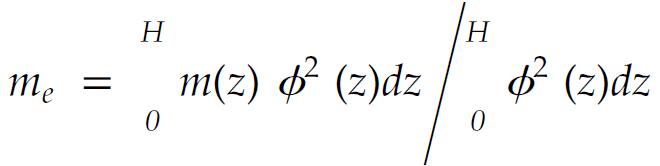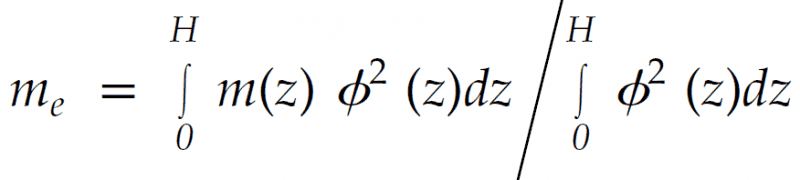# Integration of Multiple Variables

Hi There,

I'm a new member, so apologies if I've posted this in the wrong area.

I've been working through the ASME STS-1-2006 Steel Stack Standard, particularly the Vortex Shedding section.
I've come across this nasty integral which is doing my head in, and we wondering if anyone would mind taking a crack at it. (The image is not the best, but there are integral signs between the 0 and H).I need to calculate me, and have values for m, phi and H.

Any help or input is greatly appreciated.

Thanks
Daniel

SteamKing
Staff Emeritus
Homework Helper
Hi Daniel:
I've moved your thread to the Calculus forum, since it's not apparent that you are trying to solve, you know, a differential equation. The Differential Equation forum is reserved for only those types of problems.

The equation you posted seems to be lacking some math symbols, which make interpretation difficult. Can you post another image?
To make sure the image posts correctly, hit the "Preview" button in the lower right hand corner before posting.

Good Luck!

Hi SteamKing,

Thanks for the push in the right direction.
Here is another version of the equation, with the integral signsThanks!

SteamKing
Staff Emeritus
Homework Helper
You don't have to necessarily evaluate these integrals analytically. Since I assume you have values of m and φ for various values of z, you can evaluate the integrals in the numerator and denominator numerically, using Simpson's Rule for example:

http://www.intmath.com/integration/6-simpsons-rule.php

There are certain restrictions which apply to this calculation, as explained in the linked article.

Hi SteamKing,

Thanks for the link there. I've had a look at Simpson's rule before and it seems it will help.
My problem is integrating the equation itself, as I'm unsure how to do this with both "m" and "phi" being integrated with respect to z.
I looked at integration by parts, which seemed to point in the right direction, but I wasn't sure that it was entirely right for this situation.

How would you go about integrating this equation as the first step?

Thanks
Daniel

SteamKing
Staff Emeritus
Homework Helper
Hi SteamKing,

Thanks for the link there. I've had a look at Simpson's rule before and it seems it will help.
My problem is integrating the equation itself, as I'm unsure how to do this with both "m" and "phi" being integrated with respect to z.
I looked at integration by parts, which seemed to point in the right direction, but I wasn't sure that it was entirely right for this situation.

How would you go about integrating this equation as the first step?

Thanks
Daniel
There's no great mystery there.

Suppose you have a table of values of m and φ given for a range of different values of z. These are just random numbers, which may not have any bearing on the data called for by the Steel Stack Standard:

Code:
  z      m(z)      φ(z)
0.0     1.00      0.00
0.1     0.95      0.25
0.2     0.90      0.50
0.3     0.85      0.75
0.4     0.80      1.00

It's best to organize your calculations in some sort of tabular form. A spreadsheet is handy for this.

Taking the data above, we want to evaluate the two integrals from the OP to find me. For our illustration, let's also say that H = 0.4.

Setting up our calculations for forming the values of the integrands of the numerator and denominator, we can also include the Simpson's multipliers to calculate the integrals, like so:

Code:
  z      m(z)      φ(z)     φ^2(z)    m(z)*φ^2(z)    SM    Prod#1   Prod#2
0.0     1.00      0.00     0.000        0.000        1     0.000    0.000
0.1     0.95      0.25     0.063        0.059        4     0.238    0.250
0.2     0.90      0.50     0.250        0.225        2     0.450    0.500
0.3     0.85      0.75     0.563        0.478        4     1.913    2.250
0.4     0.80      1.00     1.000        0.800        1     0.800    1.000
Σ = 3.400    4.000

To be clear, Prod#1 = m(z)*φ2(z) * SM, and Prod#2 = φ2(z) * SM

Having found the sum of Prod#1 and Prod#2, the value of each integral is found by

Numerator = (1/3) * h * ΣProd#1
Denominator = (1/3) * h * ΣProd#2

For this example, the common spacing, h = 0.1, thus

∫ m(z)*φ2(z) dz ≈ (1/3) * (0.1) * 3.400 = 0.113 and
∫ φ2(z) dz ≈ (1/3) * (0.1) * 4.000 = 0.133

which makes me = 0.113 / 0.133 = .850

When you are integrating numerically, all those integration techniques, like substitution and integration by parts, are no longer necessary. You calculate the value of the integrand, apply the Simpson's multipliers, sum up the products, and then find the value of the integral.

##m_e=\frac{\int \limits_{0}^h m(z)\phi^2(z)\:dz}{\int \limits_{0}^h\phi^2(z)\:dz}##

This formula looks a lot like the centroid equation:

##\bar{x}=\frac{\int x\:dA}{\int dA}##

And for that there is a theorem called the Pappus theorem, and it says that if you rotate the area around a reference axis you'd create a volume that satisfies the following:

##V=2 \pi \bar{x} A##

##V=2 \pi \: \bar{x} \int dA##

##V=2 \pi \int x\:dA##

Now it looks more like a highschool calculus 2 problem. But without additional info we cannot analytically boil it down to something nice.

SteamKing
Staff Emeritus
Homework Helper
##m_e=\frac{\int \limits_{0}^h m(z)\phi^2(z)\:dz}{\int \limits_{0}^h\phi^2(z)\:dz}##

This formula looks a lot like the centroid equation:

##\bar{x}=\frac{\int x\:dA}{\int dA}##

And for that there is a theorem called the Pappus theorem, and it says that if you rotate the area around a reference axis you'd create a volume that satisfies the following:

##V=2 \pi \bar{x} A##

##V=2 \pi \: \bar{x} \int dA##

##V=2 \pi \int x\:dA##

Now it looks more like a highschool calculus 2 problem. But without additional info we cannot analytically boil it down to something nice.
The Second Theorem of Pappus is intended to find the volume of a figure of revolution created by revolving an area about an axis. You need to know the area and the location of its centroid relative to the axis of rotation in order to apply the theorem.

The OP's problem is clearly not related to finding the volume of a figure of revolution.

The method of numerical integration illustrated in my post #6 is a valid technique for evaluating such integrals without having to solve them analytically. One only needs to know certain values of m(z) and φ(z) for the stack in question.